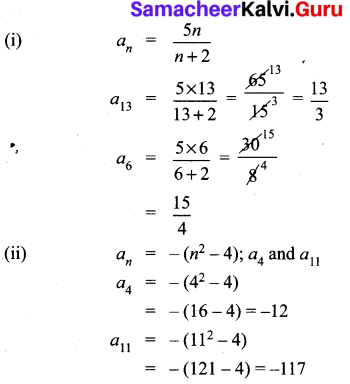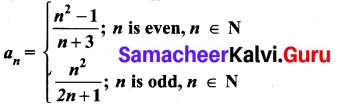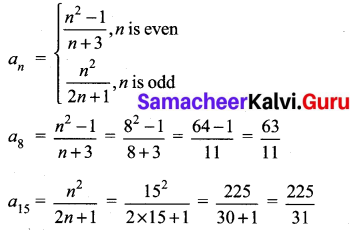# Samacheer Kalvi 10th Maths Solutions Chapter 2 Numbers and Sequences Ex 2.4

## Tamilnadu Samacheer Kalvi 10th Maths Solutions Chapter 2 Numbers and Sequences Ex 2.4

10th Maths Exercise 2.4 Samacheer Kalvi Question 1.
Find the next three terms of the following sequence.
(i) 8, 24, 72, …….
(ii) 5, 1, -3, …….
(iii) $$\frac { 1 }{ 4 }$$,$$\frac { 2 }{ 9 }$$,$$\frac { 3 }{ 16 }$$………..
Solution:
(i) 8, 24, 72…
In an arithmetic sequence a = 8,
d = t1 – t1 = t3 – t2
= 24 – 8       72 – 24
= 16 ≠ 48
So, it is not an arithmetic sequence. In a geometric sequence,
r = $$\frac{t_{2}}{t_{1}}=\frac{t_{3}}{t_{2}}$$
⇒ $$\frac{24}{8}=\frac{72}{24}$$
⇒ 3 = 3
∴ It is a geometric sequence
∴ The nth term of a G.P is tn = arn-1
∴ t4 = 8 × 34-1
= 8 × 33
= 8 × 27
= 216

t5 = 8 × 35-1
= 8 × 34
= 8 × 81
= 648

t6 = 8 × 36-1
= 8 × 35
= 8 × 243
= 1944
The next 3 terms are 8, 24, 72, 216, 648, 1944.

(ii) 5, 1, -3, …
d = t2 – t1 = t3 – t2
⇒ 1 – 5 = -3-1
-4 = -4 ∴ It is an A.P.
tn = a+(n – 1)d
t4 = 5 + 3 × – 4
= 5 – 12
= -7
15 = a + 4d
= 5 + 4 × -4
= 5 – 16
= -11
t6 = a + 5d
= 5 + 5 × – 4
= 5 – 20
= – 15
∴ The next three terms are 5, 1, -3, -7, -11, -15.

(iii) $$\frac { 1 }{ 4 }$$,$$\frac { 2 }{ 9 }$$,$$\frac { 3 }{ 16 }$$,………..
Here an = Numerators are natural numbers and denominators are squares of the next numbers
$$\frac { 1 }{ 4 }$$,$$\frac { 2 }{ 9 }$$,$$\frac { 3 }{ 16 }$$,$$\frac { 4 }{ 25 }$$,$$\frac { 5 }{ 36 }$$,$$\frac { 6 }{ 49 }$$………….

10th Maths Exercise 2.4 Question 2.
Find the first four terms of the sequences whose nth terms are given by

(i) an = n3 -2
an = n3 – 2
a1 = 13 – 2 = 1 – 2 = -1
a2 = 23 – 2 = 8 – 2 = 6
a3 = 33 – 2 = 27 – 2 = 25
a4 = 43 – 2 = 64 – 2 = 62
The four terms are -1, 6, 25 and 62

(ii) an = (-1)n+1 n(n + 1)
an = (-1)n+1 n(n + 1)
a1 = (-1)2 (1) (2) = 1 × 1 × 2 = 2
a2 = (-1)3 (2) (3) = -1 × 2 × 3 = -6
a3 = (-1)4 (3) (4) = 1 × 3 × 4 = 12
a4 = (-1)5 (4) (5) = -1 × 4 × 5 = -20
The four terms are 2, -6, 12 and -20

(iii) an = 2n2 – 6
an = 2 n2 – 6
a1 = 2(1)2 – 6 = 2 – 6 = -4
a2 = 2(2)2 – 6 = 8 – 6 = 2
a3 = 2(3)2 – 6 = 18 – 6 = 12
a4 = 2(4)2 – 6 = 32 – 6 = 26
The four terms are -4, 2, 12, 26

Ex 2.4 Class 10 Samacheer Question 3.
Find the nth term of the following sequences
(i) 2, 5, 10, 17, ……….
(ii) 0, $$\frac { 1 }{ 2 }$$, $$\frac { 2 }{ 3 }$$,…..
(iii) 3, 8, 13, 18, ………
Solution:
(i) 2, 5, 10, 17
= 12 + 1, 22 + 1, 32 + 1, 42 + 1 ……….
∴ nth term is n2+1
(ii) 0, $$\frac { 1 }{ 2 }$$,$$\frac { 2 }{ 3 }$$,………….
= $$\frac { 1-1 }{ 1 }$$,$$\frac { 2-1 }{ 2 }$$,$$\frac { 3-1 }{ 3 }$$…..
⇒ $$\frac { n-1 }{ n }$$
∴ nth term is $$\frac { n-1 }{ n }$$
(iii) 3, 8, 13, 18
a = 3
d = 5
tn = a + (n – 1)d
= 3 + (n – 1)5
= 3 + 5n – 5
= 5n – 2
∴ nth term is 5n – 2

10th Maths Exercise 2.4 In Tamil Question 4.
Find the indicated terms of the sequences whose nth terms are given by
(i) an = $$\frac { 5n }{ n+2 }$$ ; a6 and a13
(ii) an = -(n2 – 4); a4 and a11
Solution:10th Maths 2.4 Exercise Question 5.
Find a8 and a15 whose nth term isSolution:10th Maths 2.4 Question 6.
If a1 = 1, a2 = 1 and an = 2an-1 + an-2 n > 3, n ∈ N. Then find the first six terms of the sequence.
a1 = a2 = 1
an = 2an-1 + an-2
a3 = 2a3-1 + a3-2 = 2a2 + a1
= 2(1) + 1 = 3
a4 = 2a4-1 + a4-2
= 2a3 + a2
= 2(3) + 1 = 6 + 1 = 7
a5 = 2 a5-1 + a5-2
= 2a4 + a3
= 2(7) + 3 = 17
a6 = 2a6-1 + a6-2
= 2a5 + a4
= 2(17) + 7
= 34 + 7 = 41
The sequence is 1, 1, 3, 7, 17,41, …